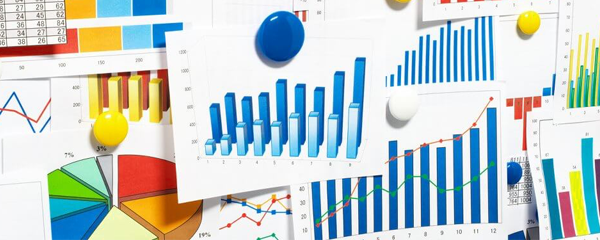SORRY OUR WEBSITE NEED JAVASCRIPT Diagram Types and Categories – DiagramArt

# Diagram Types and CategoriesWe frequently use diagrams in our daily routine without even realizing that we are actually summarizing a visual process through them. They are used at schools, offices and even at home while we read simple maps or tables and try to decipher the information that is being displayed. Therefore, it is essential to have a basic understanding of the techniques to read and decipher a diagram since they are used so frequently. Diagrams simplify tasks and convey the information in a brief and compact manner. The diagrams vary from simple to complex, depending upon the task that is being visually represented and the level of complexity that it possesses.

Diagrams are used in all fields of study in a number of different forms. The distinctive characteristic of the diagrams is the fact that they are independent of all fields of study and can be used for both quantitative as well as qualitative data according to the requirement of the task. There are numerous types of diagrams and they are not limited to a certain distinct type. They range from flow charts to bar graphs, from simple tables to complex pie charts. The type of diagram used to represent a data set or process visually depends to a large extent on the type and limitations of the data.

Some of the most common types of diagrams that are frequently used are briefly described as follows.

Flow Charts

Flow charts are frequently used diagrams that explain the various steps of a task or a process in a certain definite order. They are used in almost all educational disciplines. Moreover, they are also frequently used in offices for various project management operations as they are known for their simplicity, clarity and conciseness.

Graphs

Graphs are usually used in mathematics in order to display quantitative data. However, they can also be used to represent qualitative data. There are numerous types of graphs and they can be used depending upon the nature of the data. The categories of graphs include bar graphs, line graphs, scatter plot diagrams, pie charts and numerous others, each distinct from the other.

Triangular Diagram

Triangular diagrams are triangular structures that display information in the form of various tiers. The triangular structure is divided into a number of tiers and each tier represents a different level of hierarchy. The divisions present distinct information about each level of the hierarchy.

Timeline Diagram

Timeline diagrams are similar to the flow chart diagrams in the essential concept but they are used to represent the various steps of the tasks along with the specific time that each of the tasks will take for completion.

Activity Diagrams

Activity diagrams represent various activities along with the time that each activity takes for completion. The diagram also shows the link of activities with each other. It shows how the activities are dependent on one another.

May 07, 2015